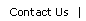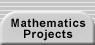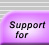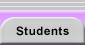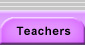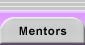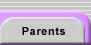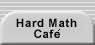# PROBLEM POSING

Students have asked me, on several occasions, "Is there any math after calculus?" These students have been given the impression that the world of mathematics is both finite and linear (the classic algebra-through-calculus sequence). They are unaware of the extraordinary variety of mathematics that either builds on or is independent of calculus. They are aware of a path, and not the full graph, of connected mathematics subjects (see Appendix). Their question also suggests that mathematics is a completed body of work. There are no new avenues to pursue or discoveries for them to make.

In any discipline, it is essential to help students understand our ignorance. They should come to appreciate the range of questions that remain open and, most importantly, the fact that countless interesting questions have yet to be thought of. Such an understanding is an invitation to join in the discussion. When teachers present mathematics as a predetermined set of facts to be transmitted, the implicit message is that students are separate from those who created the mathematics.

Mathematics research will thrive only when students see themselves as practicing, amateur mathematicians who are expected to create and work on their own questions. By its very nature, research implies a series of questions and investigations. The Making Mathematics Research Projects include multiple initial questions as well as extensions to establish this point. Good research leads to the making of connections between related findings. Without multiple results from related questions, no such broader analysis can arise.

When students begin posing their own original mathematical questions and see these questions become the focus of discussion, their perception of the subject is profoundly altered. When they get to spend time working on these questions, their ownership of the experience produces excitement and motivation.

The discussions and activities that follow will help students expand their problem-posing repertoire and promote the habit of creating new problems. However, problem-posing requires more than the mere tweaking of a pre-existing question. With structured coaching, students will also develop a greater ability to assess how interesting and productive their new questions are likely to be. As students gain in sophistication, their problems will move from aimless variations toward some clearer mathematical purpose (e.g., exploring the connection between two different areas of interest).

## CREATING NEW PROBLEMS FROM OLD ONES

Every problem is a possible seed for new problems, which come from changing, adding to, or removing one or more characteristics of the initial problem. For example, the Fibonacci sequence (1, 1, 2, 3, 5, 8, 13, 21, …) is generated by adding the previous two terms to get the next one. This is a deterministic process: the nth term is set once we choose the first two terms. The ratio between consecutive terms approaches the golden mean,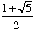, as the sequence is extended. In what ways might we alter this sequence, and what happens to the ratio in each case? For example, we can change the starting numbers, we could add more than two terms at a time, or we could change the operation being applied at each step. We can even change the rules as we calculate the sequence, e.g., let’s subtract if a coin flip shows heads and add if it shows tails. In 1960, it was found that the ratio for a random sequence such as this would still approach a particular constant value, but it was not until 1999 that computer scientist Divakar Viswanath actually figured out what that constant was (see Fibonacci at Random or New Mathematical Constant Discovered).

Most new problems are inspired either by older ones, as described above, or by contexts that direct the problem poser in a particular direction. Faced with an old question, we can try numerous changes that would lead to new investigations. The possibilities are so plentiful that a persistent student is almost assured of turning up a truly original mathematical question.

The sources of inspiration can be a well-known mathematical question, a puzzle or strategy game, or even a mundane textbook question. We can alter the conditions of these initial problems to yield interesting, open-ended research agendas. For example, a classic textbook problem supposes that a cow is tethered to the corner of a barn 12 meters long and 8 meters wide (see Figure 1). The length of the tether is 14 meters. The question asks, How much land does the cow have for grazing? A few years back, Seth, a calculus student, came to my class excitedly describing his efforts to move this problem into three dimensions. He envisioned a "space cow" tethered to the corner of a floating cube. He wondered about the volume of the region to which this cow had access. He had thought of this idea earlier in high school and now realized that he finally had the tools (integration, graphs in three dimensions, and solving systems of equations) to tackle it. The following two weeks involved the most technically challenging work that he undertook all year.Figure 1. A Cow Tethered to a Barn (aerial view)

Seth did not develop a larger theory of "cow" regions. In that respect, his questions led more to valuable problem-solving rather than to deep mathematics research. However, he was surprised by and impressed with the degree to which changing one feature of the original problem turned a trivial exercise into a difficult one (just as the introduction of randomness to the Fibonacci sequence created a problem that took decades to solve).

## WAYS TO CHANGE A PROBLEM

Having students practice creating new problems from old ones is particularly valuable if the old problems are simple enough to yield a multitude of new, solvable problems. Not only will the class get to practice the art of problem-posing, but the series of related problems will also help them build the skill of recognizing when they need to apply new technical skills toward the solution of a question. In this spirit, many of the examples that follow are further variations on the cow theme.

Share the cow problem (or another familiar problem) with your class and ask them to identify all of its numeric and geometric aspects. Then ask them to change one of those features or to change the context (setting) of the problem in some way (for example, if the cow becomes a bird or worm, you are off and running into the third dimension!). Have them write down their new problem. Finally, ask them to draw a new diagram of the situation and determine what additional mathematics they might need to solve the revised problem.

As we read and understand a problem, we become aware of the conditions that give it form. Some of these conditions are explicitly stated, while others are implied. For example, the dimensions of the barn in the cow problem are stated; here are three things that are implied: (1) the dimensions of the cow are to be ignored (i.e., we are dealing with a "point cow"), (2) we are only interested in the two-dimensional region of flat ground outside the barn, and (3) the tether is inelastic.

Below are listed some ways to change a problem to create new problems. You need not present this list to your class in its entirety, however, a posted reference list, extended each time students recognize a new problem-creation technique in their own work or in mathematics they have read, will serve as a reminder of problem-generating strategies. There are at least seven basic ways to change a problem:

• Change the numbers.
• Change the geometry.
• Change the operation.
• Change the objects under study.
• Remove a condition, or add new conditions.
• Remove or add context.
• Repeat a process.

Each of these potential changes is discussed in more detail below.

### Change the Numbers

• This is the most obvious way to change a problem. Give your students one or more problems and ask them to identify any stated or implied numbers. For example, in the cow problem, in addition to the three stated numbers, there are the following implicit conditions: the 2 dimensions of the grazing area; the 0 dimensions of the cow; the 1 dimension of the tether; the 90º angles of the barn; and the fact that there is only 1 cow with 1 tether, and that there are 0 other animals, barns, etc. We can change any of these numbers. We can also change the length and width of the barn (a and b with ab) and the length of the tether (t), which will require a case analysis, as the shape and number of regions that make up the grazing area will be different for t < a, a < t < b, b < t < a + b, and t > a + b. The geometry becomes much trickier in this final case.
• Strategy games can be a good source of research problems and often have many alterable features. Consider the game "100 or Bust" (Schielack, Chancellor, & Childs 2000):
Two players take turns rolling a die. After each roll, that player must decide whether to add the value of the roll or ten times the value of the roll to his or her score (e.g., a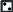can be counted as 2 or as 20). After seven rolls, the person with the highest total less than or equal to 100 is the winner. A score over 100 counts as 0.
Again, ask your class to find all the stated and assumed numbers in this game. Stated values that might be changed include the number of players, the target total (100), the number of turns, the multiples of the die result (1 or 10), and the number of dice rolled per turn. Assumed values include the number of faces on a die, the values on each face, and even the probability of each face appearing.
• When considering numerical changes to a problem, many different domains and representations can prove interesting. For example, what if we limit the domain for some variable to whole numbers, or extend it to the reals? What if we allow negative or rational numbers? What happens for particularly small or large values? What if we change base (e.g., investigate divisibility rules in base 2 instead of base 10)? Can we change a finite quantity to an infinite one or a fixed quantity to an unlimited one (e.g., now each player in "100 or bust" can take an unlimited number of turns)? What if we change from zero to a non-zero value (e.g., add width to the tether in the cow problem)?

### Change the Geometry

• Any problem with a geometric setting is ripe for new variants. The simplest problem-posing maneuver is to change the shapes involved. Different categories of shapes that suggest possible substitutions include polygons and their number of sides, regular versus non-regular polygons (Is the cow problem with different tether lengths simpler with a square barn?), convex versus non-convex figures (What if the barn were a star?), polygonal versus curvilinear figures (What if the cow were tethered to a silo with a radius of 10 meters?), and lines versus segments. Try a shape that is more general (but that includes the initial object of study, such as parallelograms rather than rectangles) or more specific (look at a subset of possibilities, such as regular solids rather than all polyhedra).
• Changes of dimension can yield exciting challenges and patterns. What if we look at pyramids rather than triangles or hyper-cubes rather than squares? What if we reduce the dimension of our problem by considering cross-sections or projections (e.g., shadows) of a higher-dimensional figure? What happens when we study graphs in coordinate spaces with three or more axes? What if our question was not about the two-dimensional area available for grazing but the one-dimensional length of the perimeter of the grazing area (so that we can buy a fence and liberate the cow from its tether)?
• The shapes we are studying may not be the only targets of our experimentation. The structure of the space in which a problem is embedded can be changed as well (e.g., What if the land on which the cow grazes is undulating?). We can transfer games played on square grids to triangular, hexagonal, semi-regular, or other tilings. We can move problems between Euclidean and non-Euclidean settings (by changing the metric). Continuous and discrete spaces (e.g., the lattice of points with integer coordinates) usually require distinct methods of solution and offer contrasting conclusions. Spaces can also be made to "wrap around" the way video arcade games often do (i.e., if you exit at an edge, you appear at the opposite one). These spaces have the same topology as the torus (which looks like the surface of a donut) and may have properties that are different from those of a standard plane. For example, the four-color theorem states that it is possible to color any planar map using at most four colors so that any two adjacent regions will have different colors. In contrast, we can draw maps on tori that require more than four colors to satisfy the same condition.
• We can add, remove, or alter the symmetry of a problem. For example, no periodic tiling of the plane has five-fold rotational symmetry. By removing the global constraint in this problem, Roger Penrose was able to create an aperiodic tiling of the plane that has local five-fold symmetries. His discovery led to significant advances in the field of crystallography.
• We can alter the location of elements in a problem. Points can be in the interior, on the boundary, or outside of a figure. For example, the cow might be tethered somewhere inside the barn, and a door could be open (or closed).

### Change the Operation

• Algebraic: We can switch between addition, subtraction, multiplication, division, exponentiation, and roots. We can also change the order of operations. Ask your class how they might change the arithmetic of the game "100 or bust"?
• Geometric: We can change between scaling, translating, rotating, and other transformations. We can construct medians rather than perpendiculars. We can trisect or n-sect rather than bisect an angle, segment, or area.
• Analytic: We can change the function involved (e.g., make it exponential rather than linear). We can also switch between equalities and inequalities, between recursive and explicit formulas, and between factored and multiplied-out expressions.
• Probabilistic: We can substitute a predictable behavior for a random one (as noted for the Fibonacci sequence, above). For example, Juancho, a fifth grader, altered the Connect the Dots problem so that the jump size was chosen randomly for each step; collecting data immediately became a more confusing challenge.

### Change the Objects Under Study

Rather than just look at real numbers, we can consider vectors, matrices, or functions (e.g., polynomials) as the operands. For example, elementary school students often discover that 2 + 2 = 2 * 2, but find no further examples (except perhaps 0 and 0). As students explore this problem with a non-integer domain, they can discover rational and irrational pairs of numbers that work. Other questions might arise; for example, what about matrices? Are there 2 x 2 matrices that multiply and add to yield equal results? Yes,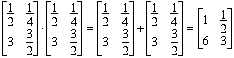and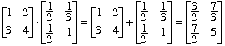are two examples. What properties do these pairs have? Are there pairs with all-integer entries (other than the trivial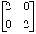)?

### Remove a Condition or Add New Conditions

• What if we add tethers but throw away the barn? What if our score for "100 or Bust" were the absolute value of the difference between our total and 100 ("100 or Close")? What if we have to declare before we roll whether to count the value of the roll once or ten-fold?
• The creation of the definition of the word semicenter (see Creating New Definitions to Create New Problems in Definitions) arose from a loosening of a condition for the center of a circle. Instead of being the point with equal-length segments to the points of a circle, a semicenter is a point with connecting segments of any length that all lie within a figure. This looser version of "center" led to a number of new questions.

### Remove or Add Context

• If a problem comes with a particular setting, we can make it abstract by removing any non-mathematical details. For example, when we begin studying taxicab geometry, the image of a street grid with buildings in each square leads us to the adoption of a new metric (a method for determining distance). One can move only sideways or up and down, because the buildings block any diagonal motion. However, those buildings also limit our ability to consider all points in the plane; we can therefore throw away the original setting but keep the metric — horizontal distance plus vertical distance — that it inspired. We are now able to consider what shapes, such as a taxicab circle, look like without worrying about the presence of buildings or other obstacles.
• Alternatively, we can add a story to an otherwise abstract problem. For example, it is easy to dissect a rectangle into four equal pieces, but when that rectangle becomes a cake and there are four kids who each want their fair share, a whole realm of new and difficult mathematics problems emerge (see "Fair Division" in COMAP  and Formulas for Fairness). Similarly, finding the area of a polygon-shaped region is easy, but determining how to cover that region with carpet of a fixed width is complicated, and finding the most efficient way to cover it with paint from a brush is more challenging still.
• You can turn each problem-posing method into a practice activity. Ask students to superimpose a story or context on an abstract problem of their choosing (e.g., a geometric construction or a system of equations they find by scanning through a textbook). Once they have created their context, they can see how it restricts or re-directs the original problem. Likewise, they can remove the story from a problem and think about variations (e.g., using negative numbers) that the setting might have implicitly discouraged.

### Repeat a Process

Iteration can lead to surprising and beautiful mathematical questions and results. For example, the famous 3x + 1 conjecture explores the fate of sequences generated by beginning with a counting number and repeatedly applying the function f(n) =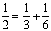(e.g., the sequence beginning with 15 continues with 46, 23, 70, 35, 106, 53, 160, 80, 40, 20, 10, 5, 16, 8, 4, 2, and 1). Although mathematicians conjecture that every starting value ultimately ends up at 1, there is no proof of this claim (see The 3x + 1 Problem and Its Generalizations). We can repeat any operation, such as squaring a number, bisecting a side, or rotating a figure, to yield ever more complicated objects or sequences for study. For additional information, see Iteration in the Mathematics Tools section.

## NEW QUESTIONS FOR OLD PROBLEMS

While we can alter many features of a problem, the most dramatic change we can impose is to change a problem’s objective. Mathematicians have a long list of questions that they are in the habit of applying to new settings; these questions, discussed below, should be introduced to beginning researchers.

### What Is the Minimum or Maximum Value Possible?

Optimizing an area, an amount, or a number of steps in a task is a common goal. For example, where along the perimeter of the barn should the farmer tether the cow in order to maximize the grazing area? Is there a shape (other than a circle) for which the attachment point is immaterial (i.e., the area is always the same, and the maximum equals the minimum)? If we can see all seven rolls in a game of "100 or Bust" before having to decide how to count each roll, what is the minimum score for which we should ever have to settle? If you could pick multiples other than 10 and 1, which two would you choose ahead of time (i.e., what values maximize the likelihood of a good score)? Sometimes, the most informative question to pose is "What is the set of objects which are all equally good (i.e., that all produce the same value)?" An understanding of these families of objects can lead one to an optimum solution.

### How Many . . . ?

Many questions have associated combinatoric lines of inquiry: How many solutions are there? How many ways are there to accomplish a mathematical task? These questions also suggest the less well defined but open-ended question, How many ways are there to solve a problem? One issue that frequently arises during efforts to enumerate possibilities is the definition of exactly what is being counted. Different definitions yield different totals, so clarity regarding what exactly makes one solution distinct from another is important (see Definitions).

### What Is the Inverse of this Question?

Charles Groetsch (1999, p. 2) defines direct problems as "those in which exactly enough information is provided . . . to carry out a well-defined stable, process leading to a unique solution." In contrast, the inverse of a direct problem may give a result and look for all the possible starting points that lead to that result. An inverse problem might also provide the beginning and end of a problem and seek the processes that could link the two. For example, an inverse of the cow problem might be, "A cow attached to a barn can graze an area of 200 square meters. What arrangements produce such an outcome?" For "100 or Bust," an inverse might be, "A player has 79 points at the end of his or her final turn; what combination of rolls might produce this result?" The name "inverse" here refers to the functional inverse: the act of undoing an operation. Problems that are the inverse of direct problems are often open-ended, just as the inverses of functions are often not simple functions themselves (i.e., they are not 1-to-1). Inverse problems naturally lead into "How many ways . . . " questions.

### What Is the Procedure?

In other words, can you find an algorithm for accomplishing a task (e.g., factoring a number or polynomial)? For example, if a cow can eat a six-inch-wide swath, what is the most efficient route she can travel in order to eat all of the grass within reach? Combinatorics problems often involve methods that count a set of mathematical objects without actually listing them. It is an interesting challenge to find a procedure for listing all the objects of a set (see the Simplex Lock Project).

### Does the Object We Have Described Actually Exist?

The answer to this question is not always clear. For example, the mathematical definition of odd perfect numbers is clear, yet no one knows whether any exist. Rather than assume solutions, we might ask, "Is it possible to make a shape (number, method, etc.) that . . . ?"

### Can We Generalize the Problem?

Once we solve a particular problem, we are tempted to explore an entire space of related problems, to look for a formula or rule that explains all of them. For example, for all cow problems, does the attachment point that maximizes grazing land always occur at a vertex or always away from one? Which games of tic-tac-toe played on an n by m board, needing r in a row to win, should be won by the first player? More abstractly, we can ask what happens when we change an exploration into the relationships between numbers into one on the relationships between element of a field or look not just at a property of addition but of binary commutative operations in general.

### What Is the Underlying Explanation for the Patterns and Structures We Encounter?

Every investigation should include these "why" questions. Why does this pattern appear in this circumstance? Why do two different situations generate the same results? Why did this change lead to that change? Mathematicians know that they are probably missing an exciting discovery if they only know that something is, but cannot say why it is so.

## PRACTICE CLASS ACTIVITIES

Your students can have a lot of fun generating problems. One way to give them a chance to practice this skill is to copy the calendar of mathematics problems from the Mathematics Teacher (NCTM) for your class. Ask each student to pick one problem and turn it into as many different new problems as possible. Have them write a list of as many explicit and implicit conditions of the problem as they can identify. Give them time to repeat this process for a variety of problems. Encourage them to pick problems involving different areas of mathematics (e.g., arithmetic, geometry, algebra).

The point of creating new problems is to create interesting problems. We do not generally know how interesting our questions are until we spend time trying to answer them. So, once your students have generated their variations, it is worthwhile to give them time to investigate those that most appeal to them. The more time students spend working on their own creations, the richer their instincts will be for the changes that lead to exciting research questions. The time spent will also help them develop their own aesthetic as problem-posing and problem-solving mathematicians.

New problems do not always require creative changes in order to produce new and interesting questions. Sometimes, changing a single number raises unexpected issues. The September 2000 Mathematics Teacher calendar included the following problem:

In Mr. Edgecomb’s mathematics class, 30 students took an exam on statistics. If the average passing grade was 84, the average failing grade was 60, and the overall average was 80, how many students passed the exam? (The answer is that 25 passed.)

One of our sophomores began to wonder if these numbers were special. So she changed the class size to 17 students. She then solved the problem by assuming that x students passed the exam. She knew that the total number of points on the 17 tests was 84x + 60(17 – x) = 80(17), which meant that x = 14.166 students passed. Since it was not possible to have a fractional part of a student, she speculated that the original averages were not exact. She recalculated what the class average would be if 14 students passed and 3 failed; the result was (14*84 + 3*60)/17 = 79.76 which rounded to 80! Now the whole class was wondering whether the numbers always allowed reasonable integral numbers of students. They also asked (but did not answer) the general question, "If the rounding does not always work, which sets of starting numbers do produce a reasonable answer?" This episode is an example of how a simple starting point can yield challenging research.

Many textbook problems like this one are designed to have "nice" answers. These exercises suggest an inverse problem: If we want integer answers to a problem, how do we find the correct starting values? Generalizing textbook problems can also be fruitful. For example, we can turn routine factoring exercises into research explorations. With the aid of a symbolic mathematics tool such as Mathematica, Maple, or the TI-89, factoring x2 – 1 and x3 – 1 can become a question about the factors of xn – 1.

Other sources that can provide the seed for new questions include simple games, books of recreational mathematics (especially those by Martin Gardner), and journals such as Quantum and The Mathematical Gazette. These materials will also introduce students to a range of unfamiliar mathematics topics.

Online sources of problems include Quantum magazine’s CyberTeasers, The Math Forum’s Problems of the Week, and Problems with a Point. The first CyberTeaser I clicked on (from January/February 2000) yielded the following challenge: "Can a number consisting of 300 ones and some number of zeros be a perfect square? Explain your answer." The solution provided assumed that the number was in base 10. Such a number would be divisible by 3 but not by 9 (because of the sum of the digits) and is therefore never going to be a perfect square, regardless of the arrangement of the digits. Many generalizations of this problem, in terms of the digits and bases involved, immediately suggest themselves.

### Clarifying a Question

Even seemingly simple changes in a problem can create an ill-defined situation. The questions below were generated by students attempting to modify the Connect the Dots research setting (see Using Research Settings and the Connect the Dots Teaching Notes for related discussions).

1. How is the pattern affected if we connect the dots with curves?
2. What if the dots are not evenly spaced around the circle? How does the placement of dots affect the shape made by the connecting lines?
3. What if the dots lie outside of the circle?
4. What if there are several dots in the middle of the circle?
5. What happens if there are concentric rings with dots?
6. What if we use a three-dimensional shape such as a sphere or a dodecahedron?
7. What if the circle is made entirely of dots?

Several of these questions offer the possibility for interesting investigations, but none, in their initial form, describe a new problem unambiguously. In question 1, we need to know the type, size, and orientation of the curves. Question 2 leaves open the matter of whether jumps are determined by dots or absolute distance. Questions 3 through 5 and 7 do not say how we are to incorporate these additional dots into the rule for jumps. Question 6, which is perhaps proposing a two-dimensional grid of dots on the surface of a solid, also needs to clarify how jumps are specified and executed in this new non-linear arrangement of dots.

Once students have proposed their variants, they should independently attempt to draw an example for each. Classes quickly discover whether a problem is clear and interpreted in a uniform manner. Once it becomes evident that a question is not clear, the original authors can attempt to rewrite their problem. Some problems may eventually prove to be quite worthwhile, while the class may never resolve other problems sufficiently to generate much interest. The process of peer review will help the students discover the need to think through the consequences of their ideas and to value careful writing.

## EVALUATING THE QUALITY OF A RESEARCH PROBLEM

Once your students are ready to work on a long-term research project, they will need to decide which questions should receive the most attention. They will not be able to work on all of the questions they propose. However, they should be encouraged to lay out a small sequence of related investigations that build on one another, which they will tackle in order. Of course, as their research proceeds, as they become more knowledgeable about their topic, and as new questions arise, their goals may shift.

For a research agenda to be a good one, several criteria need to be met:

• Students should have an intrinsic interest in the problem; if not, effort and insight are unlikely to materialize. Different questions and mathematical topics appeal to each of us. Encourage your students to develop their own (hopefully open-minded) aesthetic for mathematics. Let them know that it is appropriate to be drawn to one type of setting or another, or to prefer visual or abstract problems over others.
• Students or groups should know enough relevant mathematics to make at least some headway with their research agendas.
• Students should be able to state clearly their problems and questions.
• The questions should contain challenges but not be impossible. The worst choice for a research project is a well-known, unsolved problem. When students start talking about Goldbach’s Conjecture or some other problem that has stumped mathematicians for years, I encourage them to begin their research career with a more productive endeavor. Unsolved Mathematics Problems and The Geometry Junkyard provide interesting lists of problems not to tackle. However, while "impossible" problems should be avoided, student questions should be challenging enough so that there is no obvious solution or sure-fire method of attack.
• You and your students should assess the originality of their questions. While I do rule out Unsolved problems (with a capital U), I encourage students to tackle previously unposed problems of their own creation. The appeal of such problems is twofold: They are original to the student, and no solution is lurking in some book or journal. New problems do not come with a guarantee that they will yield interesting results, but they usually work out quite well. The fact that a student is exploring uncharted mathematical territory adds to the excitement of the experience.
Often, students will pose problems that are original to them but not to the larger mathematics community. In such cases, I ask them to avoid an early search of the literature so that they can develop a fairly deep understanding of the problem before discovering what others have done with it. By the time they embark on a literature review, they have often posed additional questions that the resources they consult do not address.
• Research that generalizes an initial setting or becomes more abstract in its treatment of that setting tends to be more satisfying than explorations that remain narrowly focused. Students should begin with more concrete and specific problems, but as they work, they should strive to solve a space of related problems rather than a single one. Searches for larger problems that encompass an original problem require creativity and encourage students to make connections between related ideas.
• The practical considerations of school life influence the suitability of a topic. A student should be able to reach at least some intermediate research objectives in the time allotted for the project. The project should also hold the promise of extensions that could easily last longer than the time allotted. Any technology required for efficient work on a problem needs to be available.

### Project Proposals

Ask students who are developing their own research questions (as opposed to using the Making Mathematics projects) to write a project proposal, which you should approve before they commit too much time to their research. A proposal should contain the following elements:

• A student’s research agenda, with a description of the problem and the main questions the student hopes to answer
• The source of the problem and why it appeals to the student
• A literature review (if appropriate) of resources that provided helpful background on the problem
• A discussion of the initial steps the student plans to take (the mathematical equivalent of an experimental design that describes the variables to be studied and the data that will be collected)

Students should use the following questions to evaluate the first draft of their project proposal:

• Have you posed a question or merely identified an area of interest?
• How original is your question?
• Is your question significant and relevant? (This is always an interesting question for pure mathematics investigations.)
• Is the question too broad or too specific to lend itself to meaningful research? If so, how can you sharpen or expand its focus?
• Can you identify the types of evidence you will need to collect during your work and what form the answers to your questions will take?
• Do you have, or can you acquire, the necessary technical background to address your question?
• Does your question allow for analysis in addition to data collection?
• Is your question realistic for the time allowed?
• Will the resources you need be available? (Answering these last four questions may require teacher guidance.)

Coming up with a good research question is important and difficult work. The more energy students invest in developing a good question, the better their research experience will be. Provide class time for your students to write "teacherly" peer reviews of one another’s project proposals, using the above questions. These reviews will help produce stronger second drafts for you to comment on. They also give students a wider audience for their work, get the class excited about one another’s questions, and provide practice in communicating technical ideas at an accessible level.

## USING RESEARCH SETTINGS

The discussions above show that students can create their own research questions by modifying old questions. Research settings — i.e., mathematical situations or objects that do not come with any initial problem statement — allow students to take the process one step further, and inspire them to ask original questions. Some of my favorite student questions have arisen from class explorations of research settings.

The Connect the Dots project is a research setting that serves as an excellent introductory experience for both middle and high school students. If you strip the problem of its questions, provide copies of the handouts, and explain the method for jumping around the circles, the designs and patterns that emerge will naturally inspire students to ask their own questions. The following questions (among dozens of others!) came from one class of fifth graders:

• What combinations of dot number and jump size make stars?
• What combinations of dot number and jump size lead to squares?
• For which dot numbers will every jump size hit every dot?
• How many regions do the segments and the circle create? (For example, a circle with 5 dots and a jump size of 1 produces 6 regions. The same circle with a jump size of 2 yields 11 regions.)
• What happens if we randomly pick a number for each jump, starting from 1 and going up to the total number of dots?

The first question about stars led to a wonderful discussion about what each student meant by the word "star." Their confusion forced them to write formal definitions of the shapes they wanted to include. Some wanted any figure with intersecting segments to be a star. Another student, because of the conjecture she was working on, defined a star as a figure such that the segments coming from a given dot connected to dots that were adjacent to each other. This dialogue naturally led to a discussion about how to write clear definitions (see Definitions).

The range of difficulty of the questions makes it possible for each student to begin work at a level appropriate to his or her skills and background. The question about squares is trivial for high school students but not for all elementary students. The question about numbers of regions is challenging at any age. Such flexibility is one of the benefits of beginning with a setting rather than a specific question.

The Mathematics Project page lists sample research settings (see Research Settings). You can also create a research setting by removing the question while leaving the description of a problem. For example, you can present your students with the Fibonacci sequence or the sequence of the powers of three and give them time to extend the lists and ask their own questions about the behaviors they note (e.g., Will there always be two odds for every even in the Fibonacci sequence?).

Sometimes a setting can be a single example of a larger phenomenon. For example, the Unit Fraction setting presents the equation. Given this lone mathematical object, what questions do your students have? Students generally note the common numerators and the multiplication problem in the denominator (2 * 3 = 6) and ask if any other equations like this exist. That question leads to wonderful discoveries and countless extensions of the setting. (Note that before students can ask a question about the object, they have to figure out what it is an example of.)

Single equations and diagrams can serve as the starting point for research. So, too, can new definitions become the seed for explorations (see Creating New Definitions to Create New Problems in Definitions). Board games and other games of strategy also provide settings that inspire mathematical research (e.g., Set). The books by Stephen Brown and Marion Walters and by Frederick Stephenson listed in the bibliography are excellent sources for problem-posing and research settings.

## BIBLIOGRAPHY

Brown, Stephen & Walters, Marion (1983). The art of problem posing. Hillsdale, NJ: Lawrence Erlbaum Associates.

Brown, Stephen & Walters, Marion (Eds.) (1993). Problem posing: Reflections and applications. Hillsdale, NJ: Lawrence Erlbaum Associates.

COMAP (1996). For all practical purposes: Introduction to contemporary mathematics. New York: W. H. Freeman and Company.

Devlin, Keith (1999). New mathematical constant discovered, MAA Online at http://www.maa.org/devlin/devlin_3_99.html.

Gardner, Martin (1986). Knotted doughnuts and other mathematical entertainments. New York: W. H. Freeman and Company.

Gardner, Martin (1988). Time travel and other mathematical bewilderments. New York: W. H. Freeman and Company.

The Geometry Junkyard is available online at http://www.ics.uci.edu/~eppstein/junkyard/open.html.

Groetsch, Charles (1999). Inverse problems. USA: Mathematics Association of America.

Lagarias, Jeff (2000). The 3x + 1 Problem and Its Generalizations. Available online at http://www.cecm.sfu.ca/organics/papers/lagarias/index.html.

The Math Forum’s Problems of the Week are available online at (http://mathforum.com/pow).

The Mathematical Gazette, information available online at http://www.m-a.org.uk/eb/periods.htm

National Council of Teachers of Mathematics (NCTM). Mathematics Teacher. Reston, Virginia. Membership and subscription information is available online at www.nctm.org or call 1-800-235-7566.

Peterson, Ivars (1996). Formulas for fairness, Science News Online at http://www.sciencenews.org/sn_arch/5_4_96/bob1.htm

Peterson, Ivars (1999). Fibonacci at random, Science News Online at http://www.sciencenews.org/sn_arc99/6_12_99/bob1.htm.

Problems with a Point is available online at http://www2.edc.org/mathproblems.

Quantum magazine, information available online at http://www.nsta.org/quantum/.

Quantum magazine’s CyberTeasers are available online at http://www.nsta.org/quantum/cyberarc.asp.

Schielack, Jane, Chancellor, Dinah, & Childs, Kimberly (2000, February). Designing questions to encourage children’s mathematical thinking. Teaching Children Mathematics, 398–402.

Stephenson, Frederick (1992). Exploratory problems in mathematics. Reston, VA: NCTM.

Unsolved Mathematics Problems is available online at http://www.mathsoft.com/asolve/index.html.

Weisstein, Eric (1999). Concise encyclopedia of mathematics CD-ROM. USA: CRC Press.

## APPENDIX

The bewildering array of topics that comprise mathematics is illustrated below by the 1991 Mathematics Subject Classifications, which is used to organize the Zentralblatt-MATH database of abstracts of mathematics journal articles. Sharing this list with your students should provide a sense of how much more there is to explore mathematically. Many of the headings will be meaningless, but you should encourage your students to note the few that they recognize. In the full classification scheme, each heading has many subheadings.

For an alternative activity that is more fun and interactive than reading this list, you can have students click through the entries of Eric Weisstein’s Concise Encyclopedia of Mathematics CD-ROM, sections of Alexander Bogomolny’s web site (http://www.cut-the-knot.com/content.html), or the Favorite Mathematical Constants page at http://pauillac.inria.fr/algo/bsolve/constant/constant.html. Another exploration option is The Mathematical Atlas (http://www.math.niu.edu/~rusin/known-math/), which is organized according to Mathematics Subject Classifications and provides a description of each heading (and the subheadings as well, which are not listed below) and additional discussions of selected topics for each. Ask your students to record and explain one new definition, problem, conjecture, and theorem that they learn about from the site they explore.

Mathematics Subject Classifications

00-XX General

01-XX History and biography

03-XX Mathematical logic and foundations

04-XX Set theory

05-XX Combinatorics

06-XX Order, lattices, and ordered algebraic structures

08-XX General algebraic systems

11-XX Number theory

12-XX Field theory and polynomials

13-XX Commutative rings and algebras

14-XX Algebraic geometry

15-XX Linear and multilinear algebra; matrix theory (finite and infinite)

16-XX Associative rings and algebras

17-XX Nonassociative rings and algebras

18-XX Category theory and homological algebra

19-XX K-theory

20-XX Group theory and generalizations

22-XX Topological groups and Lie groups

26-XX Real functions

28-XX Measure and integration

30-XX Functions of a complex variable

31-XX Potential theory

32-XX Several complex variables and analytic spaces

33-XX Special functions

34-XX Ordinary differential equations

35-XX Partial differential equations

39-XX Difference and functional equations

40-XX Sequences, series, and summability

41-XX Approximations and expansions

42-XX Fourier analysis

43-XX Abstract harmonic analysis

44-XX Integral transforms

45-XX Integral equations

46-XX Functional analysis

47-XX Operator theory

49-XX Calculus of variations and optimal control; optimization

51-XX Geometry

52-XX Convex and discrete geometry

53-XX Differential geometry

54-XX General topology

55-XX Algebraic topology

57-XX Manifolds and cell complexes

58-XX Global analysis and analysis on manifolds

60-XX Probability theory and stochastic processes

62-XX Statistics

65-XX Numerical analysis

68-XX Computer science

70-XX Mechanics of particles and systems

73-XX Mechanics of solids

76-XX Fluid mechanics

78-XX Optics and electromagnetic theory

80-XX Classical thermodynamics and heat transfer

81-XX Quantum theory

82-XX Statistical mechanics and structure of matter

83-XX Relativity and gravitational theory

85-XX Astronomy and astrophysics

86-XX Geophysics

90-XX Economics, operations research, programming, and games

92-XX Biology and other natural sciences; behavioral sciences

93-XX Systems theory; control

94-XX Information and communication; circuits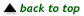Translations of mathematical formulas for web display were created by tex4ht. © Copyright 2003 Education Development Center, Inc. (EDC)